# Cofibration

(diff) ← Older revision | Latest revision (diff) | Newer revision → (diff)

A triple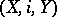, where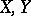are topological spaces and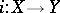is an imbedding with the following property, known as the homotopy extension property with respect to polyhedra: For any polyhedron, any mapping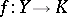and any homotopy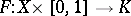with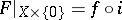there exists a homotopy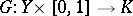such thatwhereIf this property holds with respect to any topological space, then the cofibrationis known as a Borsuk pair (in fact, the term "cofibration" is sometimes also used in the sense of "Borsuk pair" ). The spaceis called the cofibre of. The mapping cylinder construction converts any continuous mapping into a cofibration and makes it possible to construct a sequenceof topological spaces in which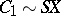(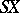is the suspension of) is the cofibre of the mapping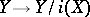— being converted into a cofibration,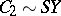is the cofibre of the mapping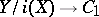, etc. Ifis a cofibration of pointed spaces, then for any pointed polyhedronthe induced sequence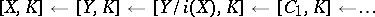is an exact sequence of pointed sets; all terms of this sequence, from the fourth onward, are groups, and from the seventh onward — Abelian groups.

How to Cite This Entry:
Cofibration. Encyclopedia of Mathematics. URL: http://encyclopediaofmath.org/index.php?title=Cofibration&oldid=13585
This article was adapted from an original article by A.F. Kharshiladze (originator), which appeared in Encyclopedia of Mathematics - ISBN 1402006098. See original article